# RBSE Maths Class 10 Chapter 2: Real Numbers Important Questions and Solutions

Chapter 2 Real Numbers Class 10 RBSE Important questions and solutions are available here. All these questions have stepwise solutions, which students can easily understand. The RBSE Class 10 important questions and solutions provided at BYJU’S helps students to improve their problem-solving skills.

Chapter 2 of RBSE Class 10 Real Numbers contains various topics and theorems such as prime factorisation method, Euclid’s division algorithm, fundamental theorem of arithmetic and so on. Students can practice enough questions on all these concepts here. For example, finding LCM and HCF of given numbers using Euclid’s division lemma (in case of two positive integers), prime factorisation method, finding the nature of decimal expansion of given numbers and proving the given number is an irrational number.

### RBSE Maths Chapter 2: Exercise 2.1 Textbook Important Questions and Solutions

Question 1: Show that the square of any positive odd integer is of the form 4q + 1, where q is any integer.

Solution:

Let a be any positive integer and b = 4.

By division algorithm,

a = bq + r; 0 ≤ r < 4 ,

That means, r = 0, 1, 2, 3

When r = 0

a = 4m

Squaring on both sides,

a² = ( 4m )²

a² = 4 ( 4m​²)

a² = 4q , where q = 4m²

It is not an odd number since it is multiple of 2.

When r = 1

a = 4m + 1

squaring both side ,

a² = ( 4m + 1)²

a² = 16m² + 1 + 8m

a² = 4 ( 4m² + 2m) + 1

a² = 4q + 1 , where q = 4m² + 2m

When r = 2

a = 4m + 2

Squaring on both sides,

a² = ​( 4m + 2)²

a² = 16m² + 4 + 16m

a² = 4 ( 4m² + 4m + 1)

a² = 4q , Where q = ​ 4m² + 4m + 1

It is not an odd number since it is multiple of 2.

When r = 3

a = 4m + 3

Squaring both hand side ,

a² = ​( 4m + 3)²

a² = 16m² + 9 + 24m

a² = 16m² + 24m ​ + 8 + 1

a² = 4 ( 4m² + 6m + 2) + 1

a² = 4q + 1 , where q = 4m² + 6m + 2

Hence, square of any positive odd integer is in the form of 4q or 4q + 1, where q is any integer.

Question 2: Use Euclid’s division algorithm to find the HCF of 210 and 55.

Solution:

210 > 55

By Euclid’s division algorithm,

210 = 55 × 3 + 45

55 = 45 × 1 + 10

45 = 10 × 4 + 5

10 = 5 × 2 + 0

Here, the remainder is 0.

Therefore, 5 is the HCF of 210 and 55.

Question 3: Use Euclid’s division algorithm to find the HCF of 420 and 130.

Solution:

420 > 130

By Euclid’s division algorithm,

420 = 130 × 3 + 30

130 = 30 × 4 + 10

30 = 10 × 3 + 0

Here, the remainder is 0.

Therefore, HCF of 420 and 130 is 10.

Question 4: Use Euclid’s division algorithm to find the HCF of 75 and 243.

Solution:

243 > 75

By Euclid’s division algorithm,

243 = 75 × 3 + 18

75 = 18 × 4 + 3

18 = 3 × 6 + 0

Here, the remainder is 0.

Therefore, 3 is the HCF of 75 and 243.

Question 5: If HCF of number 408 and 1032 is expressed in the form of 1032x – 408 × 5, then find the value of x.

Solution:

Given numbers: 408 and 1032

1032 > 408

By Euclid’s division lemma,

1032 = 408 × 2 + 216

408 = 216 × 1 + 192

216 = 192 × 1 + 24

192 = 24 × 8 + 0

Here, the remainder is 0.

Therefore, 24 is the HCF of 408 and 1032.

According to the given,

1032x – 408 × 5 = 24

1032x – 2040 = 24

1032x = 2040 + 24

1032x = 2064

x = 2064/1032 = 2

Hence, the value of x = 2.

### RBSE Maths Chapter 2: Exercise 2.2 Textbook Important Questions and Solutions

Question 6: Express each of the following numbers as a product of its prime factors.

(i) 468 (ii) 945 (iii) 20570

Solution:

(i) Prime factorization of 468: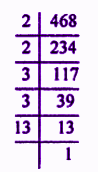468 = 2 × 2 × 3 × 3 × 13 = 22 × 32 × 13

(ii) Prime factorisation of 945: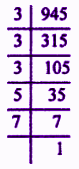945 = 3 × 3 × 3 × 5 × 7 = 33 × 5 × 7

(iii) Prime factorisation of 20570: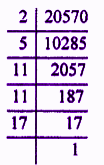20570 = 2 × 5 × 11 × 11 × 17 = 2 × 5 × 112 × 17

Question 7: Find the LCM and HCF of 96 and 404 and verify that HCF × LCM = Product of the two numbers.

Solution:

Prime factorisation of 96 and 404 is: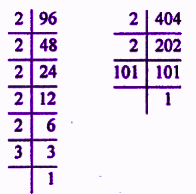96 = 2 × 2 × 2 × 2 × 2 × 3 = 25 × 3

404 = 2 × 2 × 101 = 22 × 101

LCM = 25 × 3 × 101 = 9696

HCF = 22 = 4

LCM × HCF = 9696 × 4 = 38784

Now, 96 × 404 = 38784

Therefore, LCM × HCF = Product of the two numbers

Question 8: Find the LCM and HCF of 90 and 144 and verify that HCF × LCM = Product of the two numbers.

Solution:

Prime factorisation of 90 and 144 is: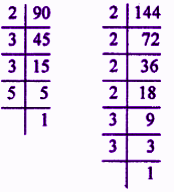90 = 2 × 3 × 3 × 5 = 2 × 32 × 5

144 = 2 × 2 × 2 × 2 × 3 × 3 = 24 × 32

LCM = 24 × 32 × 5 =720

HCF = 2 × 32 = 18

LCM × HCF = 720 × 18 = 12960

Product of 90 and 144 = 90 × 144 = 12960

Therefore, LCM × HCF = Product of the two given numbers.

Question 9: Find the LCM and HCF of the following integers by applying the prime factorisation method.

24, 15 and 36

Solution:

Prime factorisation of 24, 15 and 36 is: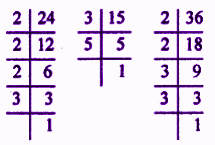24 = 2 × 2 × 2 × 3 = 23 × 3

15 = 3 × 5

36 = 2 × 2 × 3 × 3 = 22 × 32

Therefore,

LCM = 23 × 32 × 5 = 360

HCF = 3

Question 10: Find the LCM and HCF of the following integers by applying the prime factorisation method.

6, 72 and 120

Solution:

Prime factorisation of 6, 72 and 120 is: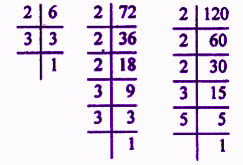6 = 2 × 3

72 = 2 × 2 × 2 × 3 × 3 = 23 × 32

120 = 2 × 2 × 2 × 3 × 5 = 23 × 3 × 5

Therefore,

LCM = 23 × 32 × 5 = 360

HCF = 2 × 3 = 6

Question 11: Find the LCM and HCF of the following integers by applying the prime factorisation method.

40, 36 and 126

Solution:

Prime factorisation of 40, 36 and 126 is: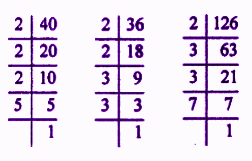40 = 2 × 2 × 2 × 5 = 23 × 5

36 = 2 × 2 × 3 × 3 = 22 × 32

126 = 2 × 3 × 3 × 7 = 2 × 32 × 7

Therefore,

LCM = 23 × 32 × 5 × 7 = 2520

HCF = 2

Question 12: In a seminar number of participants in Hindi, English and Mathematics are 60, 84 and 108, respectively. If an equal number of participants of the same subject are sitting in each room, then find the least number of rooms required.

Solution:

Number of participants in each room will be the HCF of 60, 84 and 108.

By prime factorisation,

60 = 2 × 2 × 3 × 5 = 22 × 3 × 5

84 = 2 × 2 × 3 × 7 = 22 × 3 × 7

108 = 2 × 2 × 3 × 3 × 3 = 22 × 33

HCF(60, 84, 108) = 22 × 3 = 12

Thus, 12 participants can be seated in each room

Required number of rooms = Total number of participants/ Number of participants in each room

= (60 + 84 + 108)/12

= 252/12

= 21

Therefore, required number of rooms = 21

### RBSE Maths Chapter 2: Exercise 2.3 Textbook Important Questions and Solutions

Question 13: Prove that 5 – √3 is irrational.

Solution:

Let 5 – √3 be a rational number.

Therefore, 5 – √3 = a/b, where a and b are two integers and b ≠ 0.

5 = √3 + a/b

√3 = 5 – a/b

√3 = (5b – a)/b

Here, 5, a and b are integers.

So, (5b – a)/b is a rational number.

√3 is also a rational number.

This is the contradiction to the fact that √3 is an irrational number.

Therefore, our assumption that 5 – √3 is a rational number is wrong.

Thus, 5 – √3 is an irrational number.

Hence proved.

Question 14: Prove that 3√2 is an irrational number.

Solution:

Let 3√2 be a rational number.

Therefore, 3√2 = a/b, where a and b are co-prime integers and b ≠ 0.

√2 = a/3b

Here, 3, a and b are integers.

So, a/3b is a rational number.

√2 is also a rational number.

This is the contradiction to the fact that √2 is an irrational number.

Therefore, our assumption that 3√2 is a rational number is wrong.

Thus, 3√2 is an irrational number.

Hence proved.

Question 15: If p and q are positive prime numbers, then prove that √p + √q is irrational.

Solution:

Let √p + √q be a rational number.

Therefore, √p + √q = a/b, where a, b are coprime and b ≠ 0.

√p = (a/b) – √q

Squaring on both sides,

(√p)2 = (a/b – √q)2

p = a2/b2 + q – (2a/b)√q

(2a/b)√q = a2/b2 + q – p

(2a/b)√q = [a2 + (q – p)b2] / b2

√q = [a2 + (q – p)b2] / b2 × (b/2a)

√q = [a2 + (q – p)b2] / 2ab

Here, a, b are integers.

So, [a2 + (q – p)b2] / 2ab is a rational number.

Therefore, √q is also a rational number.

This is the contradiction to the fact that √q is irrational.

Thus, our assumption that √p + √q is a rational number is wrong.

Therefore, √p + √q is an irrational number.

Hence proved.

### RBSE :Maths Chapter 2: Exercise 2.4 Textbook Important Questions and Solutions

Question 16: Without actually performing the long division method, state whether the following rational numbers will have a terminating decimal expansion or a non-terminating recurring decimal expansion.

(i) 17/6 (ii) 7/80

Solution:

(i) 17/6 = 17/ (2 × 3)

Denominator is not of the form 2m × 5n.

Therefore, 17/6 has non-terminating recurring decimal expansion.

(ii) 7/80 = 7/ (2 × 2 × 2 × 2 × 5) = 7/(24 × 51)

Denominator is of the form 2m × 5n.

Therefore, 7/80 has terminated decimal expansion.

Question 17: Write down the decimal expansion of the rational number 13/125 and state whether this is terminating.

Solution:

13/125 = 13/(5 × 5 × 5) = 13/53 = 13/ (20 × 53)

Denominator is of the form 2m × 5n.

Therefore, 13/125 has the terminating decimal expansion.

13/125 = 13/53

Multiply and divide by 23 to make the power of 10 in the denominator.

13/125 = (13 × 23) / (23 × 53)

= (13 × 8)/ (10)3

= 104/1000

= 0.104

Therefore, the decimal expansion of 13/125 is 0.104.

Question 18: Write down the decimal expansion of the rational number 14588/625 and state whether this is terminating.

Solution:

14588/625 = 14588/(5 × 5 × 5 × 5) = 14588/54 = 14588/ (20 × 54)

Denominator is of the form 2m × 5n.

Therefore, 14588/625 has the terminating decimal expansion.

14588/625 = 14588/54

Multiply and divide 24 to make the power of 10 in the denominator.

14588/625 = (14588 × 24) / (24 × 54)

= (14588 × 16)/ (10)4

= 233408/10000

= 23.3408

Therefore, the decimal expansion of 14588/625 is 23.3408.

Question 19: Write down the decimal expansion of the rational number 49/500 and state whether this is terminating.

Solution:

49/500 = 49/(2 × 2 × 5 × 5 × 5) = 49/(22 × 53)

Denominator is of the form 2m × 5n.

Therefore, 49/500 has the terminating decimal expansion.

49/500 = 49/(22 × 53)

Multiply and divide 2 to make the power of 10 in the denominator.

49/500 = (49 × 2) / (23 × 53)

= (98)/ (10)3

= 98/1000

= 0.098

Therefore, the decimal expansion of 49/500 is 0.098.

Question 20: For the below decimal expansion, decide whether it is rational or not. If it is rational, then write the note on prime factors of its denominator.

$$\begin{array}{l}27.\bar{142857}\end{array}$$

Solution:

$$\begin{array}{l}27.\bar{142857} = 27.142857142857142857…\end{array}$$

The decimal expansion of this number is non-terminating and recurring.

So, it can be written in the form p/q where q ≠ 0.

Hence, it is a rational number.

Also, it is possible that the prime factorisation of the denominator contains other prime factors other than 2 and 5.

For more practice questions on rational and irrational numbers, students can refer them here.

### RBSE Maths Chapter 2: Additional Important Questions and Solutions

Question 1: Sum of powers of prime factors of 196 is:

(a) 1

(b) 2

(c) 4

(d) 6

Solution:

Prime factorisation of 196 is: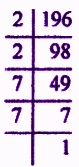196 = 2 × 2 × 7 × 7 = 22 × 72

Sum of powers of prime factors = 2 + 2 = 4

Question 2: If two numbers are written in the form m = pq3 and n = p3q2, then HCF of m, n whereas p, q are prime numbers is:

(a) pq

(b) pq2

(c) p2q2

(d) p3q3

Solution:

Given,

m = pq3 and n = p3q2

HCF is the product of least power of common factors in the prime factorisation.

HCF = pq2

Question 3: HCF of 95 and 152 is:

(a) 1

(b) 19

(c) 57

(d) 38

Solution:

Prime factorisation of 95 and 152 is:

95 = 5 x 19

152 = 23 x 19

HCF(95, 152) = 19

Question 4: Product of two numbers is 1080 and their HCF is 30 then their LCM is:

(a) 5

(b) 16

(c) 36

(d) 108

Solution:

We know that,

HCF x LCM = Product of two given numbers

30 × LCM = 1080 (given)

LCM = 1080/30

LCM = 36

Question 5: Decimal expansion of the number 441/ (22 × 57 × 72) is

(a) terminating

(b) non-terminating recurring

(c) terminating and non-terminating both

(d) not a rational number

Solution:

Given,

441/ (22 × 57 × 72)

Denominator is not in the form 2m × 5n.

Since power of 7 also exists.

Therefore, the decimal expansion is non-terminating recurring.

Question 6: In the decimal expansion of number 43/(22 × 53) after how many decimal places will terminate?

(a) 1

(b) 2

(c) 3

(d) 4

Solution:

43/ (22 × 53)

Multiply and divide by 2 to make the denominator in terms of power of 10.

(43 × 2)/ (22 × 53 × 2) = 86/(10)3

= 86/1000

= 0.086

Therefore, the decimal expansion will terminate after 3 places.

Question 7: The lowest number, when multiplied by √27 gives a natural number, will be

(a) 3

(b) √3

(c) 9

(d) 3√3

Solution:

√27 = √(3 × 3 × 3)

= 3√3

Multiply with √3,

= 3√3 × √3

= 3 × 3

= 9

Therefore, √3 is the required number to be multiplied.

Question 8: If HCF = LCM for two rational numbers, then numbers should be

(a) composite

(b) equal

(c) prime

(d) co-prime

Solution:

If HCF and LCM of two rational numbers are equal, then the numbers must be equal.

Question 9: If LCM of a and 18 is 36 and HCF of a and 18 is 2, then value of a is:

(a) 1

(b) 2

(c) 5

(d) 4

Solution:

Given,

LCM(a, 18) = 36

HCF(a, 18) = 2

We know that,

LCM × HCF = Product of the two numbers

36 × 2 = a × 18

a = 72/18

a = 4

Question 10: If n is a natural number, then unit digit in 6n – 5n is:

(a) 1

(b) 6

(c) 5

(d) 9

Solution:

We know that the unit digit of power of 6 is always 6.

For example 62 = 36, 63 = 216, ….

Also, the unit digit if power of 5 is always 5.

For example 52 = 25, 53 = 125, 54 = 625,…

Difference between 6 and 5 is 1.

Difference between 62 and 52: 36 – 25 = 11

Therefore, if n is a natural number, then the unit digit in 6n – 5n is 1.

Question 11: If p/q (q ≠ 0) is a rational number, then what condition applies for q whereas p/q is a terminating decimal?

Solution:

Given,

p/q (q ≠ 0) is a rational number and p/q has a terminating decimal.

The required condition is “prime factor of q will be in the form 2m x 5n where m, n is non-negative integers”.

Question 12: Simplify (2√45 + 3√20)/2√5 and find whether it is a rational or irrational number.

Solution:

(2√45 + 3√20)/2√5 = [2√(3 × 3 × 5) + 3√(2 × 2 × 5)] / 2√5

= (2 × 3√5 + 3 × 2√5)/2√5

= √5(6 + 6)/2√5

= 12/2

= 6

Hence, it is a rational number.

Question 13: Prove that any positive odd integer is of the form 4q + 1 or 4q + 3 where q is any integer.

Solution:

Let a be any positive odd integer and b = 4.

By applying Euclid division lemma for a and b,

a = 4q + r

Where 0 ≤ r < 4 and q is any integer.

r = 0, 1, 2, 3

When r = 0,

a = 4q + 0

⇒ a = 4q

It is an even number.

When r = 1,

a = 4q + 1

When r = 2,

a = 4q + 2

It is an even number.

When r = 3,

a = 4q + 3

When r = 4,

a = 4q + 4

It is an even number.

Therefore, any positive odd integer is of the form 4q + 1, or 4q + 3.

Hence proved.

Question 14: Prove that product of two consecutive positive integers is divisible by 2.

Solution:

Let n and (n + 1) be the two consecutive positive integers.

Product of both integers = n(n + 1) = n2 + n

or

= [(n + 1) – 1](n + 1)

= (n + 1)2 – (n + 1)

If (n + 1) is an even number, then (n + 1)2 is also an even number.

And, the difference between two even numbers is always an even number.

If (n + 1) is an odd number, then (n + 1)2 is also an odd number.

And, the difference between two odd numbers is always an even number.

Thus, the product of two consecutive positive integers is divided by 2.

Hence proved.

Question 15: Find the largest number which is divided by 2053 and 967, left remainder as 5 and 7 respectively.

Solution:

Given that, the remainder by dividing 2053 is 5.

So, 2053 – 5 = 2048 is exactly divisible by the required number.

Also, given that the remainder by dividing 967 is 7.

So, 967 – 7 = 960 is exactly divisible by the required number.

Prime factorisation of 2048 and 960 is:

2048 = 2 × 2 × 2 × 2 × 2 × 2 × 2 × 2 × 2 × 2 × 2 = 211

960 = 2 × 2 × 2 × 2 × 2 × 2 × 3 × 5 = 26 × 3 × 5

HCF of 2048 and 960 = 26 = 64

Therefore, 64 is the required number.

Question 16: A rectangular verandah is 18 m 72 cm long and 13 m 20 cm. Square tiles of the same dimensions are used to cover it. Find the least number of such tiles.

Solution:

Given,

Length of varanda = 18 m 72 cm = (1800 + 72) cm = 1872 cm

Breadth of varanda = 13 m 20 cm = (1300 + 20) cm = 1320 cm

1872 = 2 × 2 × 2 × 2 × 3 × 3 × 13 = 24 × 32 × 13

1320 = 2 × 2 × 2 × 3 × 5 × 11 = 23 × 3 × 5 × 11

Area of one square tile = HCF(1872, 1320)

= 23 × 3

= 8 × 3

= 24

Area of varanda = LCM (1872, 1320)

= 24 × 32 × 5 × 11 × 13

= 102960

Required number of tiles = Area of varanda/ Area of one square tile

= 102960/24

= 4290

Question 17: Prove that 2/√7 is an irrational number.

Solution:

Let 2/√7 be a rational number.

Therefore, 2√7 = a/b, where a and b are co-prime integers and b ≠ 0.

1/√7 = a/2b

Here, 2, a and b are integers.

So, a/2b is a rational number.

1/√7 is also a rational number.

This is the contradiction to the fact that 1/√7 is an irrational number.

Therefore, our assumption that 2/√7 is a rational number is wrong.

Thus, 2/√7 is an irrational number.

Hence proved.

Question 18: What can you say about the prime factors of the denominator of the rational number 34.12345?

Solution:

34.12345 = 3412345/100000

This of the form p/q, q ≠ 0.

Thus, it is a rational number.

q = 100000 = (10)5 = (2 × 5)5 = 25 × 55

This is of the form 2m × 5n.

Question 19: Find the HCF of 68 and 119.

Solution:

Prime factorisation of 68 and 119 is:

68 = 2 × 2 × 17 = 22 × 17

119 = 7 × 17

HCF(68, 119) = 17

Question 20: If the product of two numbers is 525 and their HCF is 5, then find their LCM.

Solution:

Given,

Product of two numbers = 525

HCF = 5

We know that,

LCM × HCF = Product of the two numbers

LCM × 5 = 525

LCM = 525/5

LCM = 105

Question 21: HCF and LCM of two integers are 12 and 336 respectively. If one integer is 48, then find another integer.

Solution:

Given,

HCF of two integers = 12

LCM of two integers = 336

One of the integers = 48

Let a be another integer.

We know that,

LCM × HCF = Product of the two numbers

336 × 12 = 48 × a

4032 = 48 × a

a = 4032/48

a = 84

Therefore, 84 is the required integer.

Question 22: Prove that √3 is an irrational number.

Solution:

Let √3 is a rational number.

Thus, √3 = a/b, where a and b are co-prime integers.

√3b = a

Squaring on both sides,

3b2 = a2 ….(i)

If 3 is a factor of a2, then 3 is also a factor of a.

Let a = 3 × m, where m is an integer.

Squaring on both sides,

a2 = (3 × m)2

a2 = 9 × m2 ….(ii)

From (i) and (ii),

3b2 = 9 × m2

b2 = 3 × m2

If 3 is a factor of b2, then 3 is also a factor of b.

Therefore, 3 is the factor of both a and b.

This is the contradiction to that fact that a and b are co-prime.

Therefore, our assumption that √3 is a rational number is wrong.

Thus, √3 is an irrational number.

Hence proved.

Question 23: Find the HCF of integers 375 and 675 by the prime factorisation method.

Solution:

Prime factorisation of 375 and 675 is: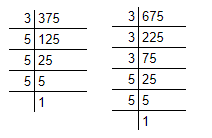375 = 3 × 5 × 5 × 5 = 3 × 53

675 = 3 × 3 × 3 × 5 × 5 = 33 × 52

HCF of 375 and 675 = 3 × 52 = 75